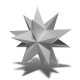# Math factoid of the day: 55

Friday 16 June 2017This is six years old. Be careful.

55 is in the Fibonacci sequence:

1 1 2 3 5 8 13 21 34 55 ...

55 is a triangular number:

1 + 2 + 3 + 4 + 5 + 6 + 7 + 8 + 9 + 10 = 55

It is the largest number that is both Fibonacci and triangular.

It is also a Kaprekar number:

55² = 3025 and 30+25 = 55

» 3 reactionsFor all that, Sammy Hagar is precluded from vehicular travel at this velocity.I'd like to see a proof that no larger Fibonacci numbers are triangular! (The linked wiki page also doesn't have any reference for that that I can see)@Chris: here's the proof: http://www.fq.math.ca/Scanned/27-2/ming.pdf I just don't understand it! :)